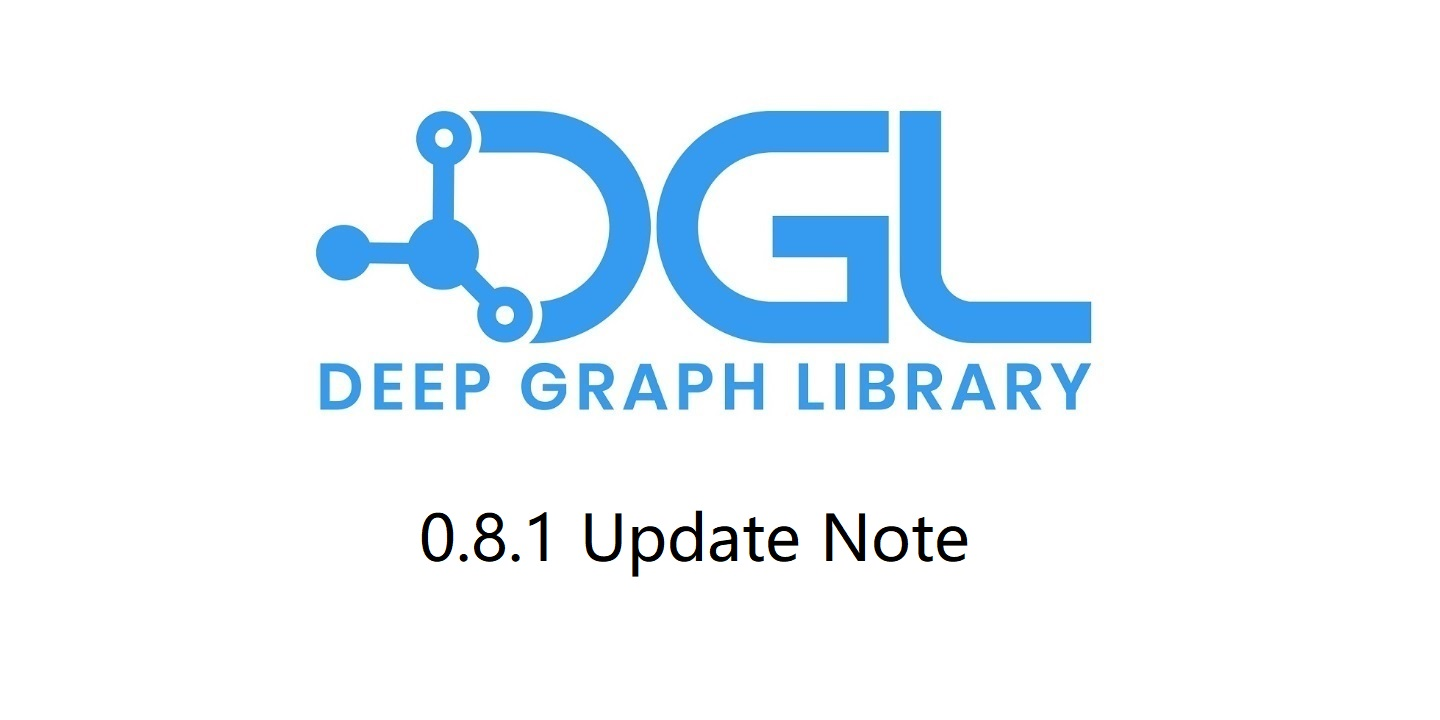## Blog Details

Home / Blog Details## Grouped Reversible Residual Connection for GNNs

We added a new module GroupRevRes introduced in Training Graph Neural Networks with 1000 Layers. It can wrap any GNN module with grouped, reversible and residual connection (example code below).

import dgl
import torch

class GNNLayer(torch.nn.Module):
def __init__(self, in_size, dropout=0.2):
super(GNNLayer, self).__init__()
# Use BatchNorm and dropout to prevent gradient vanishing
# In particular if you use a large number of GNN layers
self.norm = torch.nn.BatchNorm1d(in_size)
self.conv = dgl.nn.GraphConv(in_size, in_size)
self.dropout = torch.nn.Dropout(dropout)

def forward(self, g, x):
x = self.norm(x)
x = self.dropout(x)
return self.conv(g, x)

g = ... # some graph
x = torch.randn(g.num_nodes(), 32)
reversible_conv = dgl.nn.GroupRevRes(GNNLayer(32 // 4), 4)     # 4 groups
y = reversible_conv(g, x)  # forward


Developer Recommendation: The GroupRevRes module is reversible, meaning the backward propagation does not require storing forward activations. It can significantly reduce memory usage of GNNs, making it possible to train a very deep GNN with up to 1000 layers on a single commodity GPU.

## Laplacian Positional Encoding

We added a new data transform module LaplacianPE first introduced in Benchmarking Graph Neural Networks. It computes Laplacian positional encoding for a graph. Besides data transform module, we also provide a functional API. See the example of usage below:

import dgl

# data transform
dataset = dgl.data.CoraGraphDataset(
transform=dgl.transforms.LaplacianPE(k=2, feat_name='PE'))
g = dataset  # positional encodings will be generated as an ndata
pe = g.ndata['PE']
# functional API
pe = dgl.laplacian_pe(g, k=2)


Developer Recommendation: Laplacian positional encoding improves the expressive power of GNNs by using k-smallest non-trivial Laplacian eigenvectors as additional node features.

## Random Walk Positional Encoding

We added a new data transform module RandomWalkPE introduced in Graph Neural Networks with Learnable Structural and Positional Representations. It computes random-walk-based positional encoding for a graph. Besides data transform module, we also provide a functional API. See the example of usage below:

import dgl

# data transform
dataset = dgl.data.CoraGraphDataset(
transform=dgl.transforms.RandomWalkPE(k=2, feat_name='PE'))
g = dataset  # positional encodings will be generated automatically
pe = g.ndata['PE']
# Functional API
pe = dgl.random_walk_pe(g, k=2) # functional API


Developer Recommendation: Random walk positional encoding improves the expressive power of GNNs by using the landing probabilities of a node to itself in 1, 2, …, K steps as additional node features.

## GraphSAINT Samplers

We added a new sampler SAINTSampler introduced in GraphSAINT: Graph Sampling Based Inductive Learning Method. SAINTSampler provides three strategies to extract induced subgraphs from a graph — by randomly selected node sets, randomly selected edge sets or nodes reached by random walks. See an example of usage below:

import torch
import dgl

sampler = SAINTSampler(
mode='node',                      # Can be 'node', 'edge' or 'walk'
budget=200,
prefetch_ndata=['feat', 'label']  # optionally, specify data to prefetch
)

data_index = torch.arange(1000)  # 1000 mini-batches
g = dgl.data.CoraGraphDataset()
train(sg)


Developer Recommendation: GraphSAINT is one of the state-of-the-art sampling methods in the family of subgraph sampling. Compared with neighbor sampling (or node-wise sampling), subgraph sampling avoids the issue of exponential neighborhood expansion, thus saving data transmission cost and enabling mini-batch training of deeper GNNs.

## E(n) Equivariant Convolutional Layer

We added a new GNN module EGNNConv introduced in E(n) Equivariant Graph Neural Networks. It performs equivariant transformations on node embeddings and coordinate embeddings. See an example of usage below:

import dgl
import torch

g = ...  # some graph
h = torch.randn(g.num_nodes(), 10)   # node features
x = torch.randn(g.num_nodes(), 4)    # node coordinates
a = torch.randn(g.num_edges(), 2)    # edge features
conv = dgl.nn.EGNNConv(10, 10, 10, 2)
h, x = conv(g, h, x, a)


Developer Recommendation: GNNs with the capability of equivariant transformations have wide application in real-world structure data that have coordinates (e.g., molecules, point clouds, etc.). EGNN simplified previous attempts and proposed a design that is equivariant to rotations, translations, reflections and permutations on N-dimensional coordinates while considering both node features and node coordinates.

## Principal Neighbourhood Aggregation Layer

We added a new GNN module PNAConv introduced in Principal Neighbourhood Aggregation for Graph Nets. The code below shows an example of usage:

import dgl
import torch

g = ...  # some graph
x = torch.randn(g.num_nodes(), 10)  # node features
conv = dgl.nn.PNAConv(10, 10,
aggregators=['mean', 'max', 'sum'],
scalers=['identity', 'amplification'],
delta=2.5)
h = conv(g, x)


Developer Recommendation: Principal Neighbourhood Aggregation (PNA) improves the expressive power of a GNN by combining multiple aggregation functions with degree-scalars, thus making it a state-of-the-art baseline for many graph classification tasks.

## Survey

If there are papers for which you want to have DGL implementations or you have other feedback and suggestions, you could fill in this survey.